## Ask Your Teacher A 51-kg pole vaulter running at 11 m/s vaults over the bar. Her speed when she is above the bar is 1.1 m/s. Neglect air res

Question

Ask Your Teacher A 51-kg pole vaulter running at 11 m/s vaults over the bar. Her speed when she is above the bar is 1.1 m/s. Neglect air resistance, as well as any energy absorbed by the pole, and determine her altitude as she crosses the bar.

in progress 0
6 months 2021-08-17T11:52:45+00:00 1 Answers 0 views 0

6.11 N

Explanation:

Let,

m= mass of vaulter

Vi initial speed =11 m/s

Vf speed on pole (final) =1.1 m/s

Yi = initial height (when on ground)=o m

Yf = Height while crossing the pole =?

This problem can be solved by using conservation of energy rule;

KEi + PEi = KEf + PEf

==> 1/2 m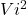+ mg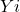= 1/2 m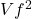+mg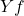Initially the altitude of vaulter is zero i-e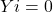==> PEi = mg= mg×0=0

==>1/2 m= /2 m+mg==> m=m+2mgcancelling m

==>=+2g==>=2g==>=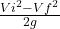==> =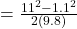==>=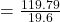=6.11N